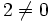# Orientable manifold

This article defines a property of manifolds and hence also of topological spaces

## Definition

A manifold is said to be orientable if it possesses an orientation, viz there exists a global section of the orientation-generator sheaf (the subsheaf of the orientation sheaf whose fibre at every point is the set of generators of the stalk at that point).

By default orientable means orientable with integer coefficients. Orientability over any ring is equivalent to orientability with integer coefficients if$2 \ne 0$ in the ring; if$2 = 0$ then any manifold is orientable with respect to that ring.

However, the number of possible orientations depends on the choice of ring; for a connected orientable manifold, the number of possible orientations equals the number of invertible element in the ring of coefficients.

## Facts

• The map from the orientation-generator sheaf to the manifold is a double cover; hence if the fundamental group of the manifold does not possess a normal subgroup of index two, the manifold must be orientable. In particular, any simply connected manifold is orientable. However, the converse is not true: real projective space in odd dimensions has fundamental group of order two, but is orientable.
• Every manifold is either orientable or has an orientable double cover: this double cover is the orientation-generator sheaf itself

## Metaproperties

### Products

This property of topological spaces is closed under taking finite products

A direct product of two orientable manifolds is again orientable. In fact, a converse statement is true: a direct product of manifolds is orientable iff each is manifold.

### Covering spaces

This property of topological spaces is closed under passing to covering spaces; viz if a topological space has this property, so does any covering space of it

Any covering space of an orientable manifold is again an orientable manifold (the manifold structure is given in the usual way.

### Fiber bundles

This property of topological spaces is a fiber bundle-closed property of topological spaces: it is closed under taking fiber bundles, viz if the base space and fiber both satisfy the given property, so does the total space.
Manifold, Orientable manifold

If$E$ is a fiber bundle with base space$B$ and fiber space$F$, and if$B$ and$F$ are both orientable manifolds, then so is$E$. Note that this covers the case of direct products and covering space.

The converse is not necessarily true, but some weaker variants thereof are. Fill this in later